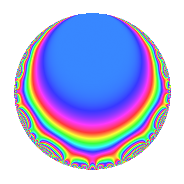# Properties

 Label 71.1.b.aLevel 71 Weight 1 Character orbit 71.b Self dual Yes Analytic conductor 0.035 Analytic rank 0 Dimension 3 Projective image $$D_{7}$$ CM disc. -71 Inner twists 2

# Related objects

## Newspace parameters

 Level: $$N$$ = $$71$$ Weight: $$k$$ = $$1$$ Character orbit: $$[\chi]$$ = 71.b (of order $$2$$ and degree $$1$$)

## Newform invariants

 Self dual: Yes Analytic conductor: $$0.0354336158969$$ Analytic rank: $$0$$ Dimension: $$3$$ Coefficient field: $$\Q(\zeta_{14})^+$$ Coefficient ring: $$\Z[a_1, a_2]$$ Coefficient ring index: $$1$$ Projective image $$D_{7}$$ Projective field Galois closure of 7.1.357911.1 Artin image size $$14$$ Artin image $D_7$ Artin field Galois closure of 7.1.357911.1

## $q$-expansion

Coefficients of the $$q$$-expansion are expressed in terms of a basis $$1,\beta_1,\beta_2$$ for the coefficient ring described below. We also show the integral $$q$$-expansion of the trace form.

 $$f(q)$$ $$=$$ $$q$$ $$-\beta_{1} q^{2}$$ $$+ ( -1 + \beta_{1} - \beta_{2} ) q^{3}$$ $$+ ( 1 + \beta_{2} ) q^{4}$$ $$+ \beta_{2} q^{5}$$ $$+ ( -1 + \beta_{1} ) q^{6}$$ $$+ ( -1 - \beta_{2} ) q^{8}$$ $$+ ( 1 - \beta_{1} ) q^{9}$$ $$+O(q^{10})$$ $$q$$ $$-\beta_{1} q^{2}$$ $$+ ( -1 + \beta_{1} - \beta_{2} ) q^{3}$$ $$+ ( 1 + \beta_{2} ) q^{4}$$ $$+ \beta_{2} q^{5}$$ $$+ ( -1 + \beta_{1} ) q^{6}$$ $$+ ( -1 - \beta_{2} ) q^{8}$$ $$+ ( 1 - \beta_{1} ) q^{9}$$ $$+ ( -1 - \beta_{2} ) q^{10}$$ $$- q^{12}$$ $$+ ( -\beta_{1} + \beta_{2} ) q^{15}$$ $$+ \beta_{1} q^{16}$$ $$+ ( 2 - \beta_{1} + \beta_{2} ) q^{18}$$ $$-\beta_{1} q^{19}$$ $$+ ( 1 + \beta_{1} ) q^{20}$$ $$+ q^{24}$$ $$+ ( \beta_{1} - \beta_{2} ) q^{25}$$ $$+ ( -1 + \beta_{1} ) q^{27}$$ $$+ ( -1 + \beta_{1} - \beta_{2} ) q^{29}$$ $$+ q^{30}$$ $$- q^{32}$$ $$-\beta_{1} q^{36}$$ $$-\beta_{1} q^{37}$$ $$+ ( 2 + \beta_{2} ) q^{38}$$ $$+ ( -1 - \beta_{1} ) q^{40}$$ $$+ \beta_{2} q^{43}$$ $$- q^{45}$$ $$+ ( 1 - \beta_{1} ) q^{48}$$ $$+ q^{49}$$ $$- q^{50}$$ $$+ ( -2 + \beta_{1} - \beta_{2} ) q^{54}$$ $$+ ( -1 + \beta_{1} ) q^{57}$$ $$+ ( -1 + \beta_{1} ) q^{58}$$ $$-\beta_{2} q^{60}$$ $$+ q^{71}$$ $$+ \beta_{1} q^{72}$$ $$+ \beta_{2} q^{73}$$ $$+ ( 2 + \beta_{2} ) q^{74}$$ $$+ ( 1 - \beta_{2} ) q^{75}$$ $$+ ( -1 - \beta_{1} - \beta_{2} ) q^{76}$$ $$+ \beta_{2} q^{79}$$ $$+ ( 1 + \beta_{2} ) q^{80}$$ $$+ ( 1 - \beta_{1} + \beta_{2} ) q^{81}$$ $$-\beta_{1} q^{83}$$ $$+ ( -1 - \beta_{2} ) q^{86}$$ $$+ ( 2 - \beta_{1} ) q^{87}$$ $$+ ( -1 + \beta_{1} - \beta_{2} ) q^{89}$$ $$+ \beta_{1} q^{90}$$ $$+ ( -1 - \beta_{2} ) q^{95}$$ $$+ ( 1 - \beta_{1} + \beta_{2} ) q^{96}$$ $$-\beta_{1} q^{98}$$ $$+O(q^{100})$$ $$\operatorname{Tr}(f)(q)$$ $$=$$ $$3q$$ $$\mathstrut -\mathstrut q^{2}$$ $$\mathstrut -\mathstrut q^{3}$$ $$\mathstrut +\mathstrut 2q^{4}$$ $$\mathstrut -\mathstrut q^{5}$$ $$\mathstrut -\mathstrut 2q^{6}$$ $$\mathstrut -\mathstrut 2q^{8}$$ $$\mathstrut +\mathstrut 2q^{9}$$ $$\mathstrut +\mathstrut O(q^{10})$$ $$3q$$ $$\mathstrut -\mathstrut q^{2}$$ $$\mathstrut -\mathstrut q^{3}$$ $$\mathstrut +\mathstrut 2q^{4}$$ $$\mathstrut -\mathstrut q^{5}$$ $$\mathstrut -\mathstrut 2q^{6}$$ $$\mathstrut -\mathstrut 2q^{8}$$ $$\mathstrut +\mathstrut 2q^{9}$$ $$\mathstrut -\mathstrut 2q^{10}$$ $$\mathstrut -\mathstrut 3q^{12}$$ $$\mathstrut -\mathstrut 2q^{15}$$ $$\mathstrut +\mathstrut q^{16}$$ $$\mathstrut +\mathstrut 4q^{18}$$ $$\mathstrut -\mathstrut q^{19}$$ $$\mathstrut +\mathstrut 4q^{20}$$ $$\mathstrut +\mathstrut 3q^{24}$$ $$\mathstrut +\mathstrut 2q^{25}$$ $$\mathstrut -\mathstrut 2q^{27}$$ $$\mathstrut -\mathstrut q^{29}$$ $$\mathstrut +\mathstrut 3q^{30}$$ $$\mathstrut -\mathstrut 3q^{32}$$ $$\mathstrut -\mathstrut q^{36}$$ $$\mathstrut -\mathstrut q^{37}$$ $$\mathstrut +\mathstrut 5q^{38}$$ $$\mathstrut -\mathstrut 4q^{40}$$ $$\mathstrut -\mathstrut q^{43}$$ $$\mathstrut -\mathstrut 3q^{45}$$ $$\mathstrut +\mathstrut 2q^{48}$$ $$\mathstrut +\mathstrut 3q^{49}$$ $$\mathstrut -\mathstrut 3q^{50}$$ $$\mathstrut -\mathstrut 4q^{54}$$ $$\mathstrut -\mathstrut 2q^{57}$$ $$\mathstrut -\mathstrut 2q^{58}$$ $$\mathstrut +\mathstrut q^{60}$$ $$\mathstrut +\mathstrut 3q^{71}$$ $$\mathstrut +\mathstrut q^{72}$$ $$\mathstrut -\mathstrut q^{73}$$ $$\mathstrut +\mathstrut 5q^{74}$$ $$\mathstrut +\mathstrut 4q^{75}$$ $$\mathstrut -\mathstrut 3q^{76}$$ $$\mathstrut -\mathstrut q^{79}$$ $$\mathstrut +\mathstrut 2q^{80}$$ $$\mathstrut +\mathstrut q^{81}$$ $$\mathstrut -\mathstrut q^{83}$$ $$\mathstrut -\mathstrut 2q^{86}$$ $$\mathstrut +\mathstrut 5q^{87}$$ $$\mathstrut -\mathstrut q^{89}$$ $$\mathstrut +\mathstrut q^{90}$$ $$\mathstrut -\mathstrut 2q^{95}$$ $$\mathstrut +\mathstrut q^{96}$$ $$\mathstrut -\mathstrut q^{98}$$ $$\mathstrut +\mathstrut O(q^{100})$$

Basis of coefficient ring in terms of $$\nu = \zeta_{14} + \zeta_{14}^{-1}$$:

 $$\beta_{0}$$ $$=$$ $$1$$ $$\beta_{1}$$ $$=$$ $$\nu$$ $$\beta_{2}$$ $$=$$ $$\nu^{2} - 2$$
 $$1$$ $$=$$ $$\beta_0$$ $$\nu$$ $$=$$ $$\beta_{1}$$ $$\nu^{2}$$ $$=$$ $$\beta_{2}\mathstrut +\mathstrut$$ $$2$$

## Character Values

We give the values of $$\chi$$ on generators for $$\left(\mathbb{Z}/71\mathbb{Z}\right)^\times$$.

 $$n$$ $$7$$ $$\chi(n)$$ $$-1$$

## Embeddings

For each embedding $$\iota_m$$ of the coefficient field, the values $$\iota_m(a_n)$$ are shown below.

For more information on an embedded modular form you can click on its label.

Label $$\iota_m(\nu)$$ $$a_{2}$$ $$a_{3}$$ $$a_{4}$$ $$a_{5}$$ $$a_{6}$$ $$a_{7}$$ $$a_{8}$$ $$a_{9}$$ $$a_{10}$$
70.1
 1.80194 0.445042 −1.24698
−1.80194 −0.445042 2.24698 1.24698 0.801938 0 −2.24698 −0.801938 −2.24698
70.2 −0.445042 1.24698 −0.801938 −1.80194 −0.554958 0 0.801938 0.554958 0.801938
70.3 1.24698 −1.80194 0.554958 −0.445042 −2.24698 0 −0.554958 2.24698 −0.554958
 $$n$$: e.g. 2-40 or 990-1000 Significant digits: Format: Complex embeddings Normalized embeddings Satake parameters Satake angles

## Inner twists

Char. orbit Parity Mult. Self Twist Proved
1.a Even 1 trivial yes
71.b Odd 1 CM by $$\Q(\sqrt{-71})$$ yes

## Hecke kernels

There are no other newforms in $$S_{1}^{\mathrm{new}}(71, [\chi])$$.